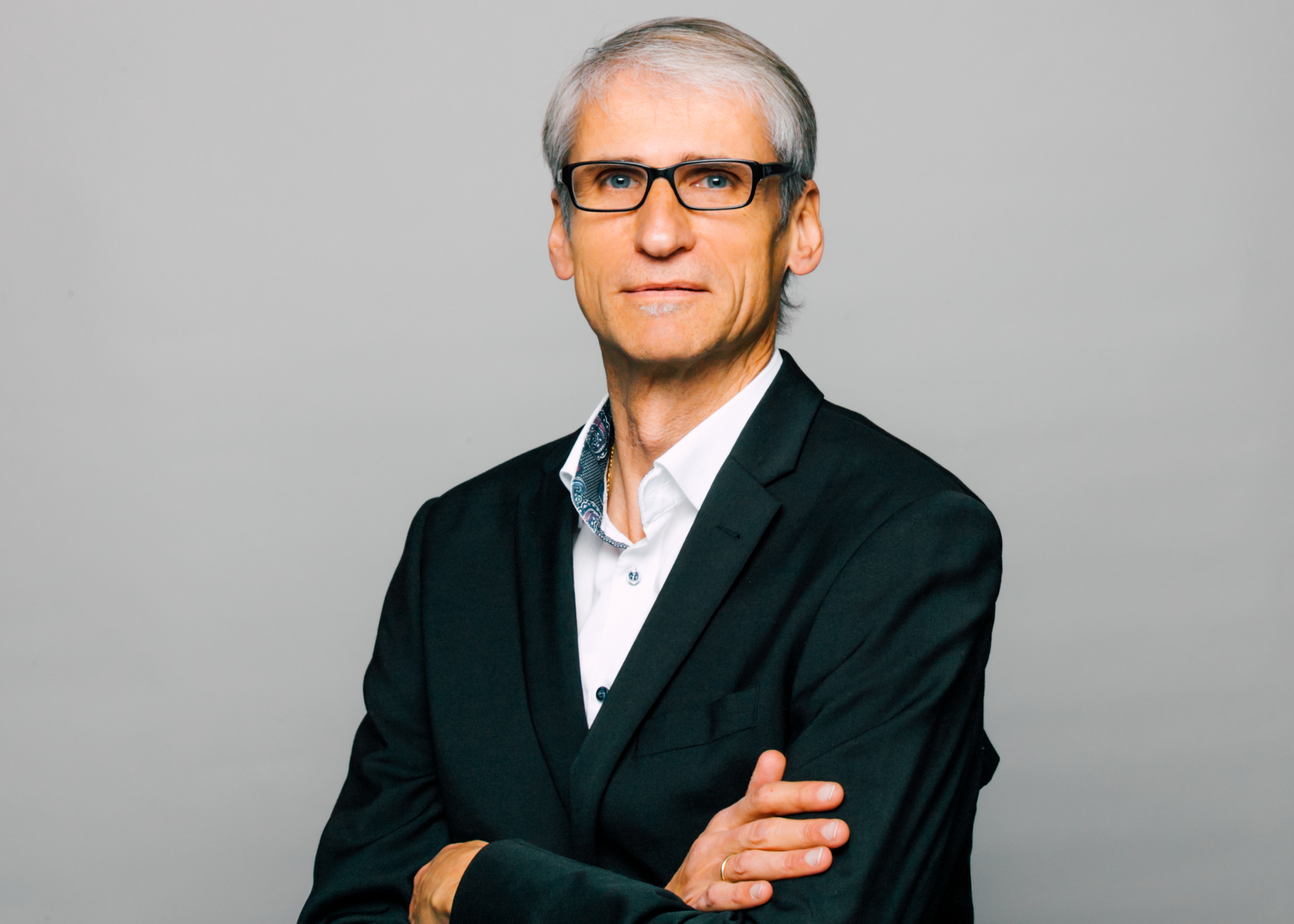# Thermodynamic cycle calculation

IGTE have been active in thermodynamics research and teaching for decades and offer their expert knowledge for services in thermodynamic cycle calculations.

Thermodynamic (cycle) processes are used to convert heat in work (right-hand heat and power processes) and to provide heat at low temperatures (left-hand refrigerating machine process) or at high temperatures (left-hand heat pump process). Today, different processes are more and more combined with each other to improve the energy efficiency for energy conversion and energy supply. As an example, the thermal recuperation of combustion engines can increase the overall efficiency of the energy conversion due to the additional use of the residual energy from exhaust gas. This is enabled by the combination of the engine process with a Clausius Rankine (CR) process and an Organic Clausius Rankine (OCR) process, respectively.

The energetic description of the operating principle of all involved system components is an element of the thermodynamic cycle calculation. For this purpose, the dependence on thermal (p, T, v) and caloric (u, h, s) state variables as well as their calculation via state equations for the used work equipment of the cycles need to be enabled. Normally, the thermodynamic cycle is calculated for the steady operating state. The conservation equations for mass and energy are solved by a suitable simulation program. The command variables such as efficiency factors, state variables and process variables can be analyzed numerically and visualized in charts. Information about cycle optimization are possible by considering entropy and exergy.

### Contact